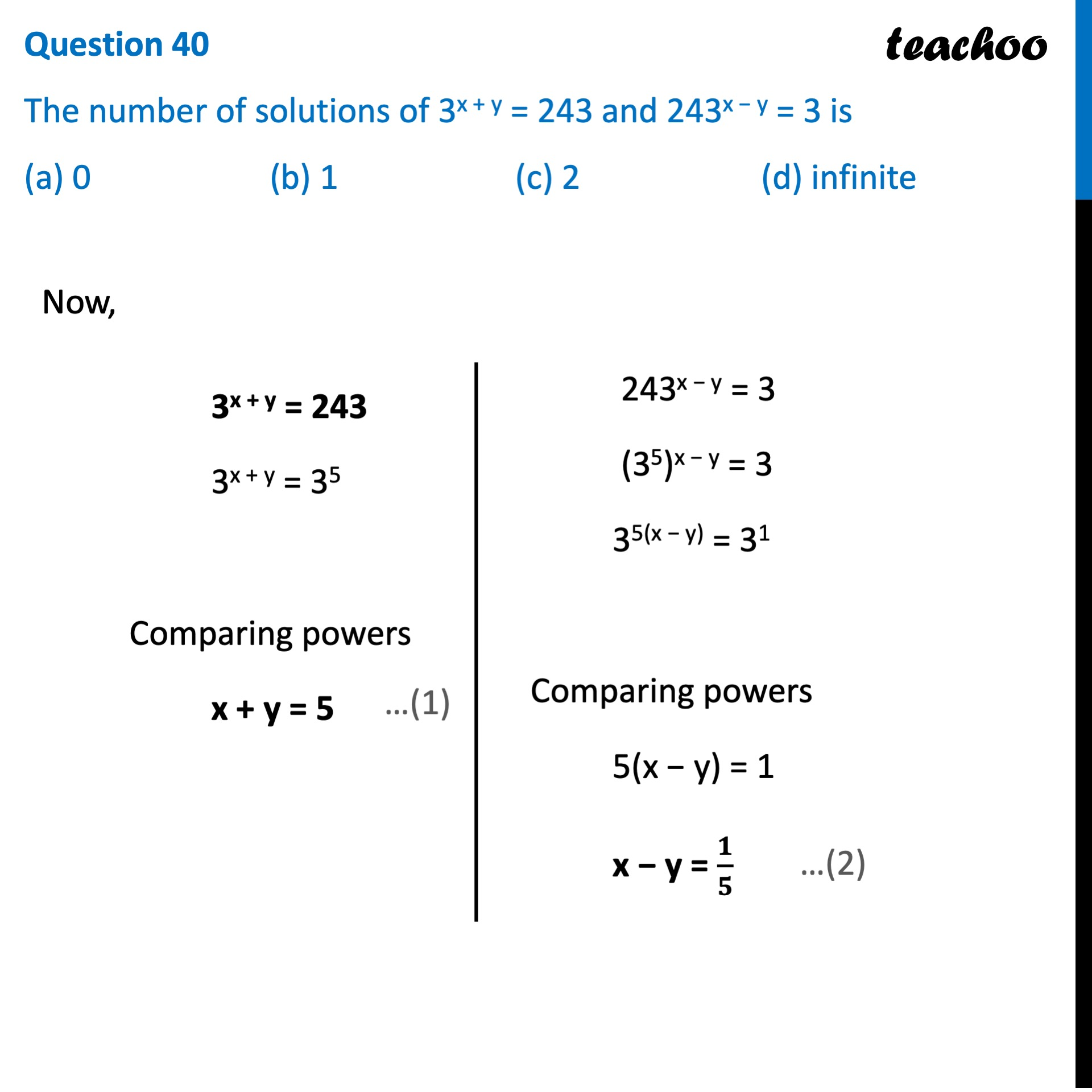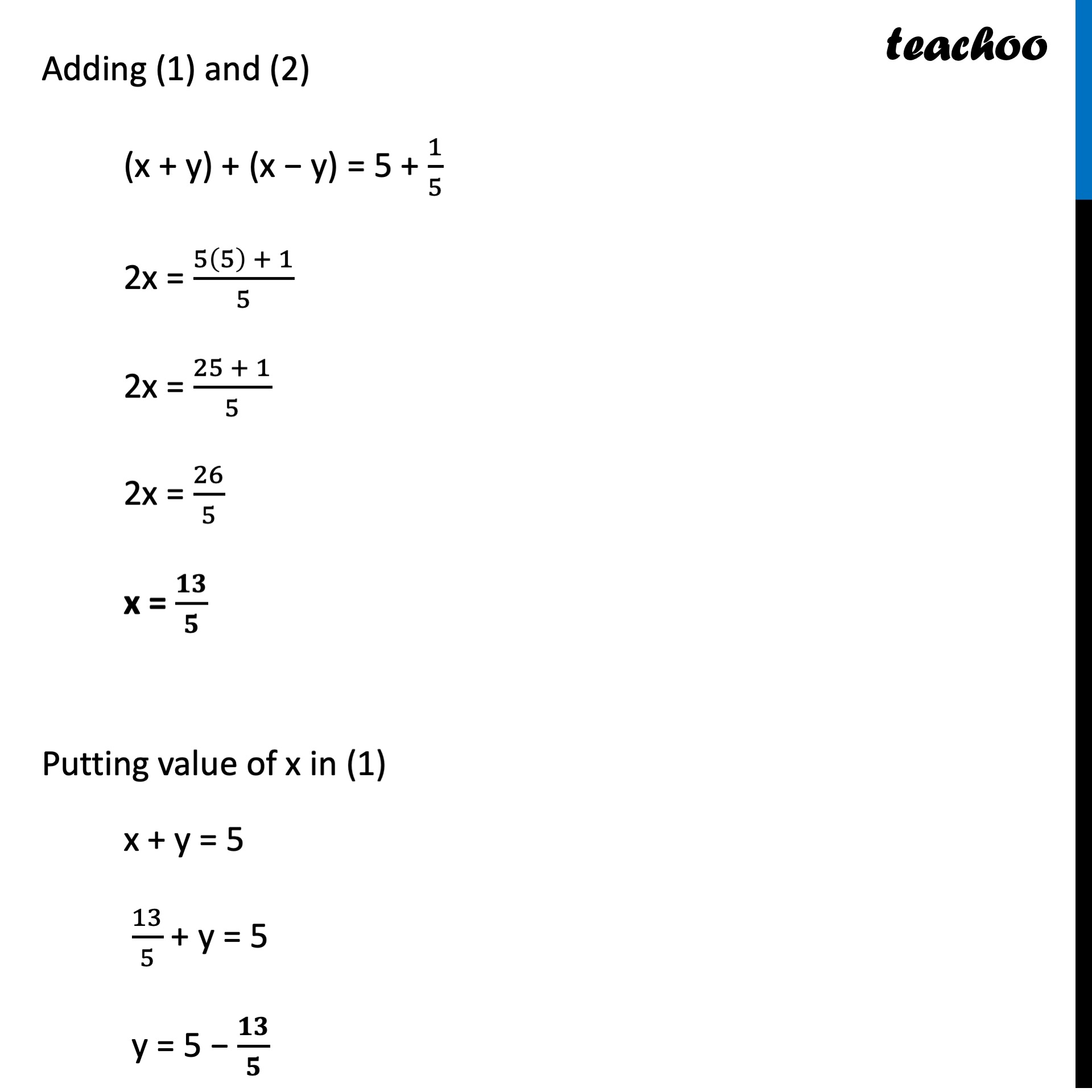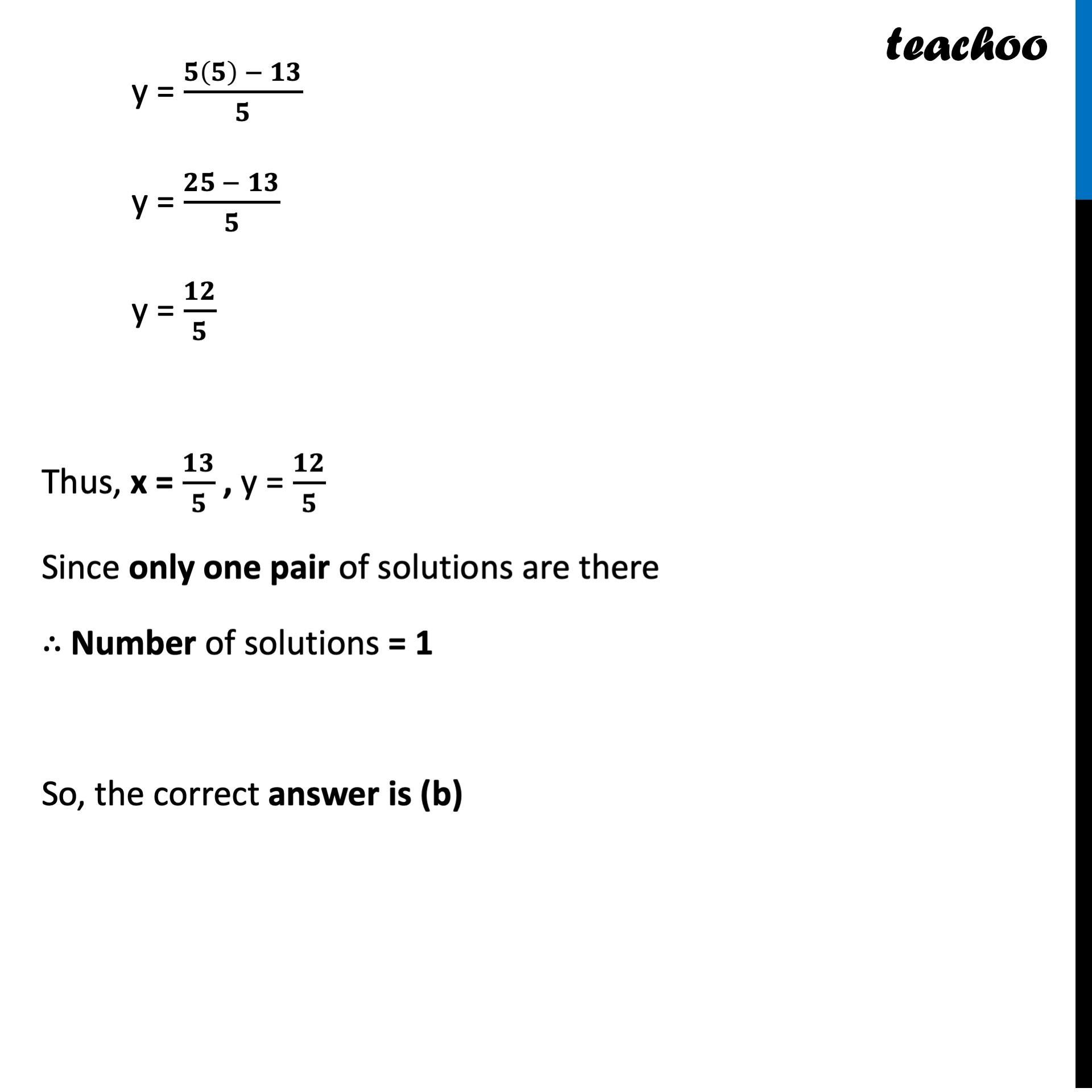CBSE Class 10 Sample Paper for 2022 Boards - Maths Standard [MCQ]

Class 10
Solutions of Sample Papers for Class 10 Boards

## (a) 0  (b) 1   (c) 2   (d) infiniteLearn in your speed, with individual attention - Teachoo Maths 1-on-1 Class

### Transcript

Question 40 The number of solutions of 3x + y = 243 and 243x − y = 3 is (a) 0 (b) 1 (c) 2 (d) infinite 3x + y = 243 3x + y = 35 Comparing powers x + y = 5 243x − y = 3 (35)x − y = 3 35(x − y) = 31 Comparing powers 5(x − y) = 1 x − y = 𝟏/𝟓 Adding (1) and (2) (x + y) + (x − y) = 5 + 1/5 2x = (5(5) + 1)/5 2x = (25 + 1)/5 2x = 26/5 x = 𝟏𝟑/𝟓 Putting value of x in (1) x + y = 5 13/5 + y = 5 y = 5 − 𝟏𝟑/𝟓 y = (𝟓(𝟓) − 𝟏𝟑)/𝟓 y = (𝟐𝟓 − 𝟏𝟑)/𝟓 y = 𝟏𝟐/𝟓 Thus, x = 𝟏𝟑/𝟓 , y = 𝟏𝟐/𝟓 Since only one pair of solutions are there ∴ Number of solutions = 1 So, the correct answer is (b)